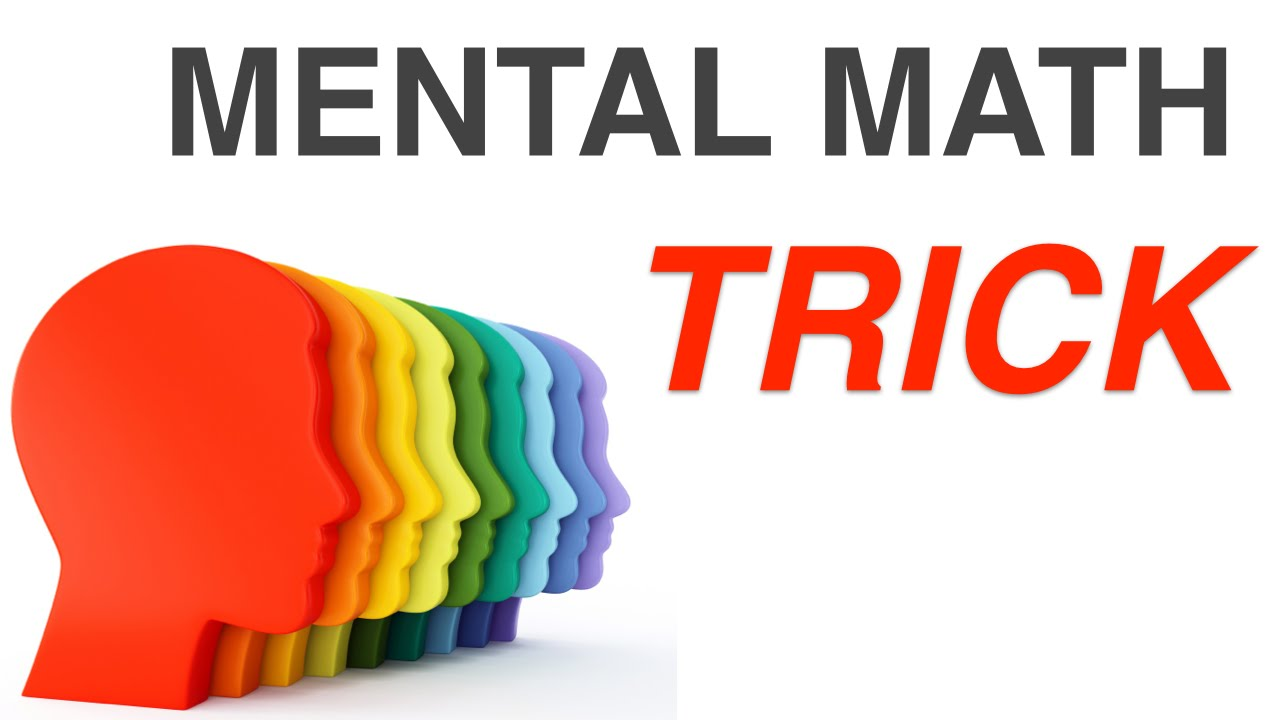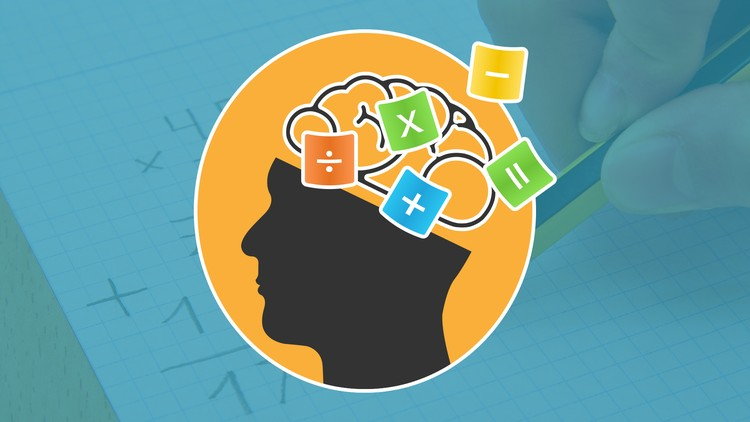# Mental calculation tricks to teach student to add fast

Sometimes, we are presented with mathematical problems. We do not have a calculator to solve them. That is when we have to resort to our mental calculation skills. Here, we offer some mental calculation tricks to make it much faster.

## Mental calculation tricks to teach students

Change the order in which we add the figures, simplify the problems, or break down an operation into several small, some tips to study. They will be very useful when you do not have a calculator at hand.

### Add and subtract from left to right

From children, we were taught to add from right to left, but when it comes to mental calculation, it is easier to do it the other way around. Thus, if we have to add 58 + 26, it is better to add 50 + 20 = 70 and then 8 + 6 = 14. We add 70 + 14 = 84 to find the solution.### Simplify the problem by changing some values

For example, to calculate 593 + 680, we can add 7 to 593 to have 600, an easier number to use. Therefore, we add 600 + 680 and get 1,280. Now, we subtract the 7 we took ‘borrowed’ before to have the final result, 1,273. It is also valid with multiplications. To find out how much 89 x 6 is, we calculate 90 x 6 and to the result (540), we subtract the ‘loan’ to get 534.

### Decompose a large problem into several small ones

The example of Gizmodo is: multiplying a number by 8 is the same as doubling it three times. So, to know how much is 12 x 8 it is enough to double three times 12: 24, 28 and 96. To multiply by 5, the trick is to multiply first by 10 and then divide by two, very simple operations. So, for example, if we have 18 x 5, we can do 18 x 10 = 180 and 180/2 = 90.

### Move the comma to calculate percentages

You are in a restaurant and the account is 112.23 dollars. You want to leave the 20 or 15% tip. How to calculate it? First make 10%, which is as easy as moving the comma (11.22 dollars). If you want to know double, 20%, multiply by two (22.44 dollars). To calculate 15%, add your half to 10%, 11.22 + 11.22/2, which gives us 16.83.

### Refresh the tricks to multiply what you learned in school

Every number multiplied by 5 ends in 5 or 0. Multiplying by 12 is ten times the number plus twice that same number (4 x 12 = 4 x 10 + 4 + 4 = 48). Multiplying by 15 is the same as multiplying by 10 and adding half (4 x 15 = 4 x 10 + 20 = 60).

### Dominate the sum

From an ingenious way to make it easier for us to add 8 and 9 to the addition tables or some general basic strategies to start … the resources on the sum are numerous! You can not miss this great game, in which you must clear all the numbers on the screen finding the two numbers that, added together, result in the number indicated.### Multiplying is very fun

To learn to multiply in a different way, do not miss this trick to calculate the product of a whole number of two figures by 11 or this online game of mental calculation, which will make the little ones have a great time.

Fractions and tricks. To understand these mathematical expressions and learn to use them easily, we recommend two resources. To pass from fraction to decimal number with denominator equal to 2, 20 or 200; Take a look at this resource. In addition, with these activities, you can learn to perform different types of operations with fractions.

### Do not stop researching

The main objective is to be able to get your own tricks. To do this, do not stop asking questions, investigating new ways, working with numbers. It will also be very helpful to motivate you to take part in mental calculation competitions or to use the best sources on the subject. It is an online method of math learning that reinforces mental calculation and teaches thinking and reasoning.

The ideal is that the student ends up understanding and assimilating the correct application of the commutative, associative and distributive properties of the numbers so they can be used and customized as they improve.